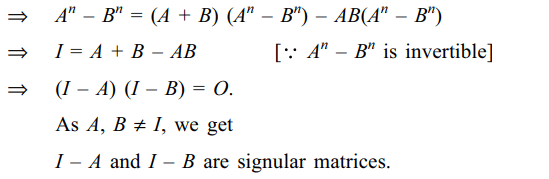## Matrices and Determinants Questions and Answers Part-4

1. Let A, B, C be three 3 * 3 matrices with entries from R such that ABC + AB + BC + AC + A + B + C = O.
a) If A commutes with B + C then A commutes with BC
b) If A commutes with BC then A commutes with B + C
c) A + I is invertible
d) All of the above

Explanation: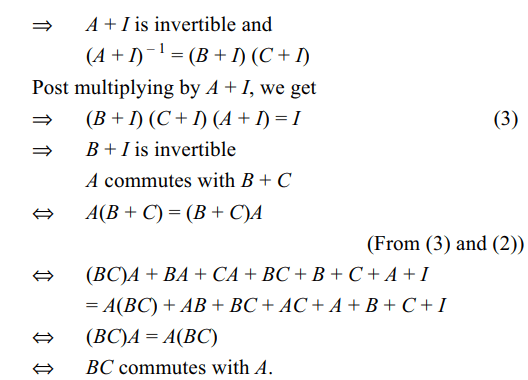2. Suppose A, B are two distinct $2 \times2$   matrices such that
$A^{2}-5A+6I=0$
$B^{2}-5B+6I=0$
then
a) $A^{3}-B^{3}=19\left(A-B\right)$
b) $A^{4}-B^{4}=65\left(A-B\right)$
c) $A^{5}-B^{5}=211\left(A-B\right)$
d) All of the above

Explanation: A3– B3 = (5A2– 6A) – (5B2– 6B)3. Let $\omega\neq 1$   be cube root of unity and let P = pij be $n \times n$  matrix such that $p_{ij}=\omega^{i+j}$    . Then $P^{2}\neq O$   when n is
a) 56
b) 55
c) 58
d) All of the above

Explanation: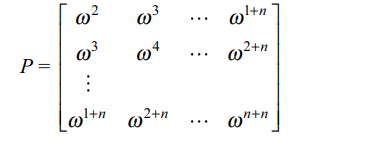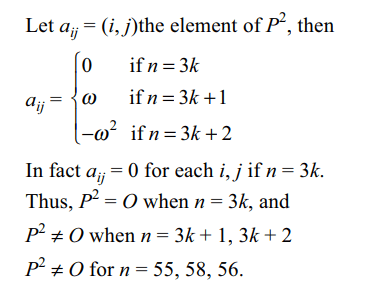4. Let $A=\left(a_{ij}\right)_{3\times 3},B=\left(b_{ij}\right)_{3\times 3}$      be two real symmetric matrices such that $A^{2}+B^{2}=O$  , then
a) AB = O
b) A = O
c) B = O
d) All of the above

Explanation:5. Let A be $4 \times4$  matrix with real entries such that determinant of every $2 \times2$  submatrix is 0, then
b) det(A) = 0
c) AX = O has infinite number of solutions
d) All of the above

Explanation: As determinant of every 2 * 2 submatrix is 0.6. Let $P=\begin{bmatrix}3 & -1 & -2 \\2 & 0 & \alpha \\3 & -5 & 0\end{bmatrix}$      , where $\alpha\epsilon R$ , Suppose $Q=\left[q_{ij}\right]$   is a matrix such that PQ = kI, where $k\epsilon R,k\neq 0$   and I is the identity matrix of order 3. If $q_{23}=\frac{k}{8}$   and det$\left(Q\right)=\frac{k^{2}}{2}$     , then
a) $\alpha=0 ,k=8$
b) $4\alpha-k+8=0$
c) det(P adj(Q))= $2^{9}$
d) Both b and c

Explanation: PQ = kI
Q = (kI) P–1 = kP–1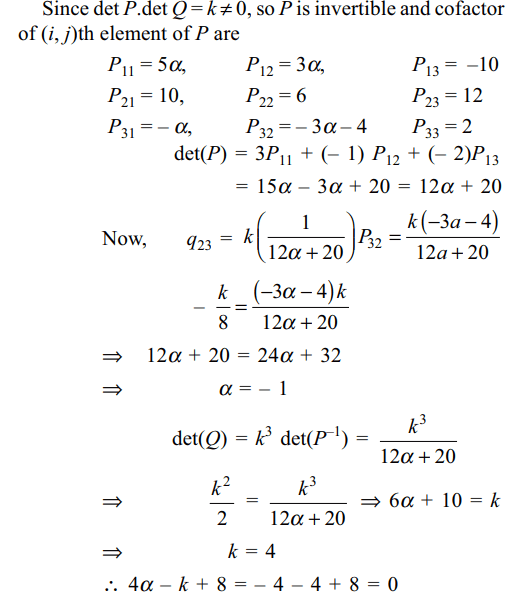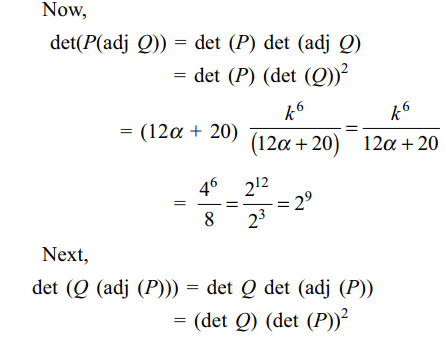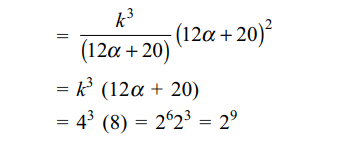7. Let M be a $2 \times2$  symmetric matrix with integer entries. Then M is invertible if
a) the product of entries in the main diagonal of M is not the square of an integer
b) the second row of M is the transpose of the first column of M
c) M is a diagonal matrix with nonzero entries in the main diagonal
d) Both a and c

Explanation: As M is a 2 × 2 symmetric matrix8. Let X and Y be two arbitrary, $3 \times3$  , nonzero, skew-symmetric matrices and Z be an arbitrary $3 \times3$  , non-zero, symmetric matrix. Then which of the following matrices is (are) skew symmetric?
a) $X^{23}+Y^{23}$
b) $X^{44}+Y^{44}$
c) $X^{4}Z^{3}-Z^{3}Y^{4}$
d) Both a and c

Explanation: X' = –X, Y' = –Y, Z' = Z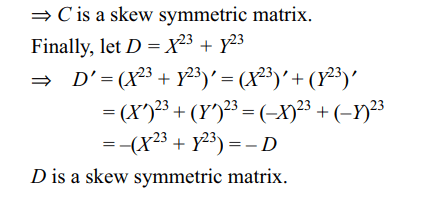9. Let A, B be two $2 \times2$  real commutative matrices, such that det $\left(A^{2}+AB+B^{2}\right)=0$     , then
a) $det \left(A-\omega B\right)=0$
b) $det \left(A-\omega^{2} B\right)=0$
c) $det \left(A+ B\right)=0$
d) Both a and b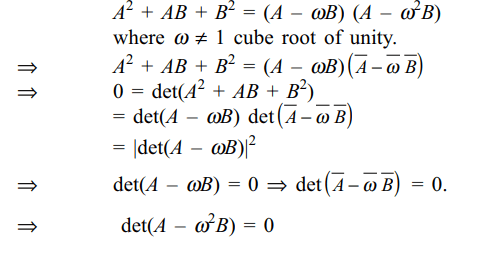10. Let A and B be two matrices different from I such that AB = BA and An – Bn is invertible for some positive integer n. If $A^{n}-B^{n}=A^{n+1}-B^{n+1}=A^{n+2}-B^{n+2}$        , then